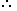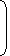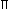# Short Tricks to Solve Problems on AreaFUNDAMENTAL CONCEPTS

1. Results on Triangles:
1. Sum of the angles of a triangle is 180°.
2. The sum of any two sides of a triangle is greater than the third side.
3. Pythagoras Theorem:In a right-angled triangle, (Hypotenuse)2 = (Base)2 + (Height)2.
4. The line joining the mid-point of a side of a triangle to the positive vertex is called the median.
5. The point where the three medians of a triangle meet, is called centroid. The centroid divided each of the medians in the ratio 2 : 1.
6. In an isosceles triangle, the altitude from the vertex bisects the base.
7. The median of a triangle divides it into two triangles of the same area.
8. The area of the triangle formed by joining the mid-points of the sides of a given triangle is one-fourth of the area of the given triangle.
1. The diagonals of a parallelogram bisect each other.
2. Each diagonal of a parallelogram divides it into triangles of the same area.
3. The diagonals of a rectangle are equal and bisect each other.
4. The diagonals of a square are equal and bisect each other at right angles.
5. The diagonals of a rhombus are unequal and bisect each other at right angles.
6. A parallelogram and a rectangle on the same base and between the same parallels are equal in area.
7. Of all the parallelogram of given sides, the parallelogram which is a rectangle has the greatest area.

IMPORTANT FORMULAE

1. 1.   Area of a rectangle = (Length x Breadth).Length =Areaand Breadth =Area. Breadth Length

2.   Perimeter of a rectangle = 2(Length + Breadth).

2. Area of a square = (side)2 =(diagonal)2.
3. Area of 4 walls of a room = 2 (Length + Breadth) x Height.
4. 1.   Area of a triangle =x Base x Height.2.   Area of a triangle = s(sa)(sb)(sc)
where abc are the sides of the triangle and s =(a + b + c).

 3.   Area of an equilateral triangle = 3 x (side)2. 4
 4.   Radius of incircle of an equilateral triangle of side a = a . 23
 5.   Radius of circumcircle of an equilateral triangle of side a = a . 3
 6.   Radius of incircle of a triangle of areaand semi-perimeter r =. s
5. 1.   Area of parallelogram = (Base x Height).2.   Area of a rhombus =x (Product of diagonals).3.   Area of a trapezium =x (sum of parallel sides) x distance between them.
6. 1.   Area of a circle =R2, where R is the radius.2.   Circumference of a circle = 2R.
 3.   Length of an arc = 2R, whereis the central angle. 360
 4.   Area of a sector = 1 (arc x R) =R2. 2 360
7. 1.   Circumference of a semi-circle =R.
 2.   Area of semi-circle =R2 . 2

### Area – General Questions

1. The ratio between the length and the breadth of a rectangular park is 3 : 2. If a man cycling along the boundary of the park at the speed of 12 km/hr completes one round in 8 minutes, then the area of the park (in sq. m) is:
 A. 15360 B. 153600 C. 30720 D. 307200

Explanation:

 Perimeter = Distance covered in 8 min. =12000 x 8m = 1600 m. 60

Let length = 3x metres and breadth = 2x metres.

Then, 2(3x + 2x) = 1600 or x = 160.Length = 480 m and Breadth = 320 m.Area = (480 x 320) m2 = 153600 m2.

An error 2% in excess is made while measuring the side of a square. The percentage of error in the calculated area of the square is:
 A. 2% B. 2.02% C. 4% D. 4.04%

Explanation:

100 cm is read as 102 cm.A1 = (100 x 100) cm2 and A2 (102 x 102) cm2.

(A2 – A1) = [(102)2 – (100)2]

= (102 + 100) x (102 – 100)

= 404 cm2.Percentage error =404 x 100% = 4.04% 100 x 100

#### The ratio between the perimeter and the breadth of a rectangle is 5 : 1. If the area of the rectangle is 216 sq. cm, what is the length of the rectangle?

Correct! Wrong!

#### The percentage increase in the area of a rectangle, if each of its sides is increased by 20% is

Correct! Wrong!

#### A rectangular park 60 m long and 40 m wide has two concrete crossroads running in the middle of the park and rest of the park has been used as a lawn. If the area of the lawn is 2109 sq. m, then what is the width of the road

Correct! Wrong!

#### A towel, when bleached, was found to have lost 20% of its length and 10% of its breadth. The percentage of decrease in area is:

Correct! Wrong!

#### A man walked diagonally across a square lot. Approximately, what was the percent saved by not walking along the edges?

Correct! Wrong!

QUIZ ON PROBLEMS OF AREA
EXCELLENT